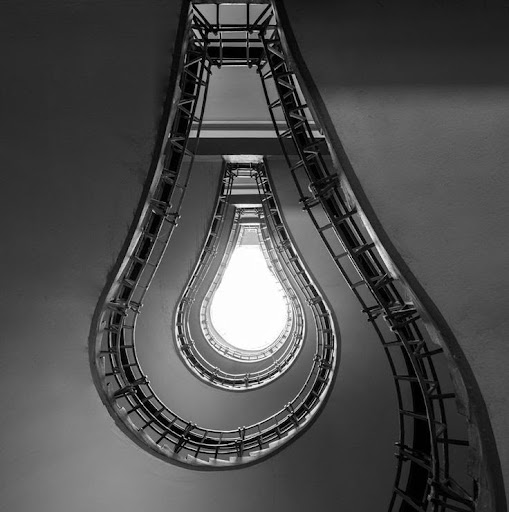ShaDiliX - 2 years ago 163
Java Question

Constructor Chain

Why do we use this form? (it's on a course on plural sight for Java)

public MathEquation(char opCode)
{
this.opCode = opCode;
}

public MathEquation(char opCode , double leftVal , double rightVal)
{
this(opCode);
this.leftVal = leftVal;
this.rightVal = rightVal;
}

and why not this?

public MathEquation(char opCode , double leftVal , double rightVal)
{
this.opCode = opCode
this.leftVal = leftVal;
this.rightVal = rightVal;
}JB Nizet